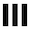##dwitter.net

+ New dweet
`function u(t) {`
```} // 122/140 ```

Please log in (or register) to post a dweet (copy-paste the code somewhere safe to save it meanwhile)

``` u(t) is called 60 times per second. t: Elapsed time in seconds. S: Shorthand for Math.sin. C: Shorthand for Math.cos. T: Shorthand for Math.tan. R: Function that generates rgba-strings, usage ex.: R(255, 255, 255, 0.5) c: A 1920x1080 canvas. x: A 2D context for that canvas. ```
remix of d/13410 by u/katkip

`function u(t) {`

```}// 139/140 ```

Please log in (or register) to post as a new dweet (copy-paste code somewhere safe to save it meanwhile).

• u/katkip
Nice
• u/sigveseb
Pretty af
• u/Purpzie
Shortened by 5 characters: `if(t<2)with(x)globalAlpha=.7,fillRect(0,0,1,1),rotate(Math.PI*.8),translate(-450,-700),drawImage(c,0,0,1e3,1e3),translate(-900,-310)`

``` u(t) is called 60 times per second. t: elapsed time in seconds. c: A 1920x1080 canvas. x: A 2D context for that canvas. S: Math.sin C: Math.cos T: Math.tan R: Generates rgba-strings, ex.: R(255, 255, 255, 0.5) ```